# 《R的极客理想—工具篇》—— 1.7　R语言的高质量图形渲染库Cairo

+关注继续查看

### 1.7　R语言的高质量图形渲染库Cairo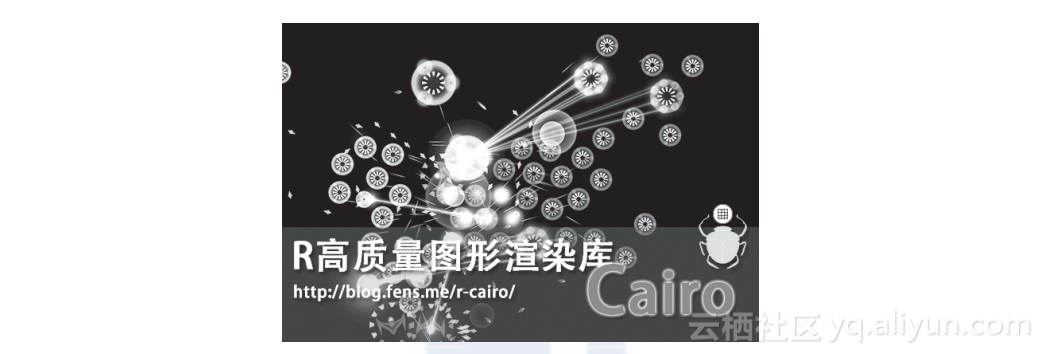R语言不仅在统计分析和数据挖掘领域计算能力强大，它在数据可视化领域也不逊于昂贵的商业软件。当然，R在可视化上强大，其背后离不开各种开源软件包的支持，Cairo就是这样一个用于矢量图形处理的类库。Cairo可以创建高质量的矢量图形(GIF、SVG、 PDF、PostScript) 和位图(PNG、JPEG、TIFF)，同时支持在后台程序中高质量渲染！本节将介绍Cairo在R语言中的使用。

#### 1.7.1　Cairo介绍

Cairo 是一个用于图形绘图和渲染的免费库，支持复杂的 2D 的绘图功能，支持硬件加速。虽然，Cairo 是用C语言编写的，但提供多种语言的接口，允许其他语言直接调用，包括有 C++、C#、Java、Python、Perl、Ruby、Scheme、Smalltalk 等语言。Cairo发布的许可协议为 GNU Lesser General Public License version 2.1(LGPL) 或 Mozilla Public License 1.1(MPL)。

#### 1.7.2　Cairo包安装

Linux: Ubuntu 12.04.2 LTS 64bit
R: 3.0.1 x86_64-pc-linux-gnu

Cairo包在Linux Ubuntu系统中的安装过程如下：

~ sudo apt-get install libcairo2-dev  # Cairo的底层依赖库
~ sudo apt-get install libxt-dev
~ R  # 启动R程序
> install.packages("Cairo")  # 安装Cairo包

#### 1.7.3　Cairo使用

Cairo使用起来非常简单，和基础包grDevices中的函数对应。
CairoPNG: 对应grDevices:png()。
CairoJPEG: 对应grDevices:jpeg()。
CairoTIFF: 对应grDevices:tiff()。
CairoSVG: 对应grDevices:svg()。
CairoPDF: 对应grDevices:pdf()。

> library(Cairo)  # 加载Cairo包
> Cairo.capabilities()  # 检查Cairo包支持的图片格式
png   jpeg   tiff    pdf    svg     ps    x11    win raster
TRUE   TRUE  FALSE   TRUE   TRUE   TRUE   TRUE  FALSE   TRUE

1. 散点图
首先我们来画一个6000个点的散点图。
> x<-rnorm(6000)  # 随机取6000个点坐标
> y<-rnorm(6000)
> png(file="plot4.png",width=640,height=480)  # png函数
> plot(x,y,col="#ff000018",pch=19,cex=2,main = "plot")
> dev.off()
> CairoPNG(file="Cairo4.png",width=640,height=480)  # CairoPNG函数
> plot(x,y,col="#ff000018",pch=19,cex=2,main = "Cairo")
> dev.off()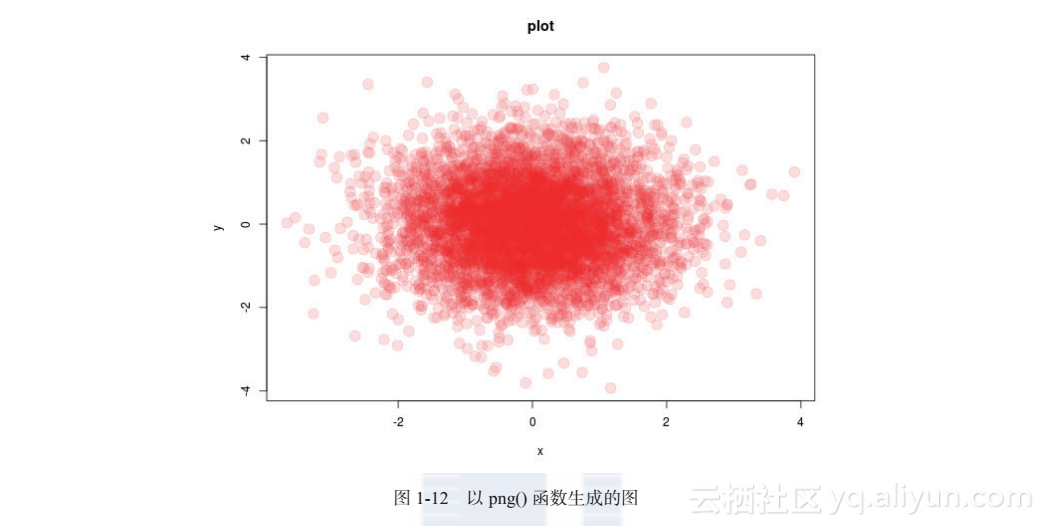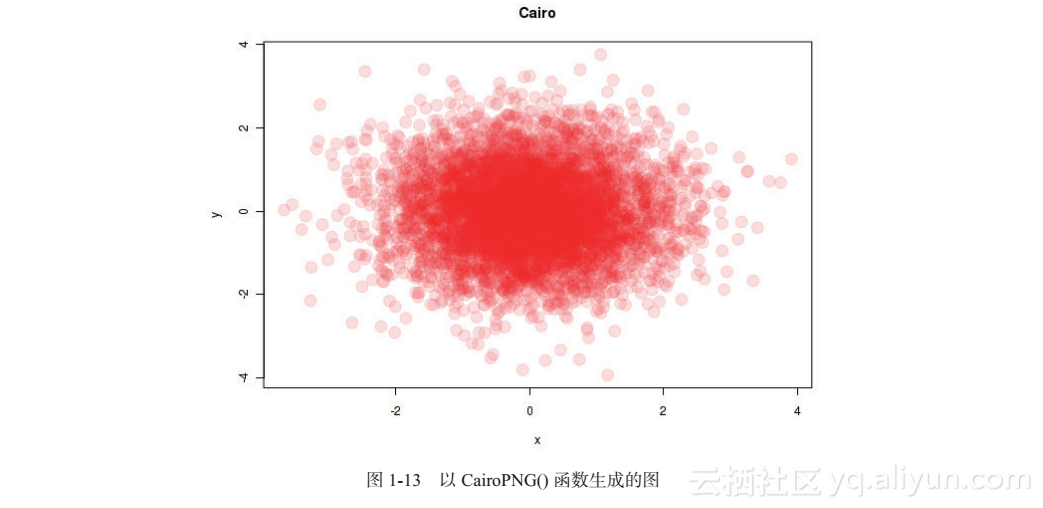SVG图形输出代码为：

> svg(file="plot-svg4.svg",width=6,height=6)
> plot(x,y,col="#ff000018",pch=19,cex=2,main = "plot-svg")
> dev.off()
> CairoSVG(file="Cairo-svg4.svg",width=6,height=6)
> plot(x,y,col="#ff000018",pch=19,cex=2,main = "Cairo-svg")
> dev.off()

1. 三维截面图
接下来，我们再画一个三维截面图，比较函数png()和CairoPNG()输出的效果。
> x <- seq(-10, 10, length= 30)
> y <- x
> f <- function(x,y) { r <- sqrt(x^2+y^2); 10 * sin(r)/r }
> z <- outer(x, y, f)
> z[is.na(z)] <- 1
> png(file="plot2.png",width=640,height=480)  # PNG图
> op <- par(bg = "white", mar=c(0,2,3,0)+.1)
> persp(x, y, z,theta = 30, phi = 30,expand = 0.5,col = "lightblue",ltheta =
120,shade = 0.75,ticktype = "detailed",xlab = "X", ylab = "Y", zlab =
"Sinc(r)",main = "Plot")
> par(op)
> dev.off()
> CairoPNG(file="Cairo2.png",width=640,height=480)
> op <- par(bg = "white", mar=c(0,2,3,0)+.1)
> persp(x, y, z,theta = 30, phi = 30,expand = 0.5,col = "lightblue",ltheta =
120,shade = 0.75,ticktype = "detailed",xlab = "X", ylab = "Y", zlab =
"Sinc(r)",main = "Cairo")
> par(op)
> dev.off()

1. 大量文字的图片
最后，我们针对包含大量文字的图片比较png()和CairoPNG()函数输出的效果。
> library(MASS)  # 加载MASS包
> data(HairEyeColor)  # 加载HairEyeColor数据集
> x <- HairEyeColor[,,1]+HairEyeColor[,,2]
> n <- 100
> m <- matrix(sample(c(T,F),n^2,replace=T), nr=n, nc=n)
> png(file="plot5.png",width=640,height=480)  # PNG图
> biplot(corresp(m, nf=2), main="Plot")
> dev.off()

> CairoPNG(file="Cairo5.png",width=640,height=480)
> biplot(corresp(m, nf=2), main="Cairo")
> dev.off()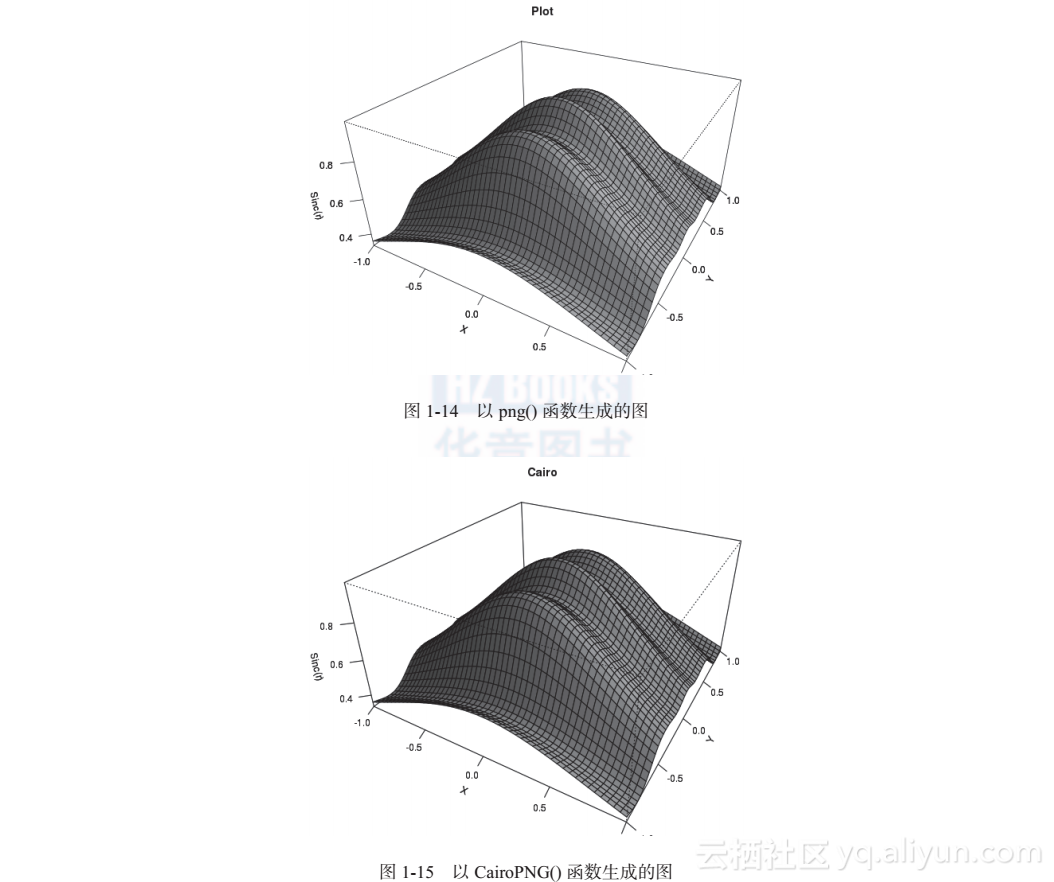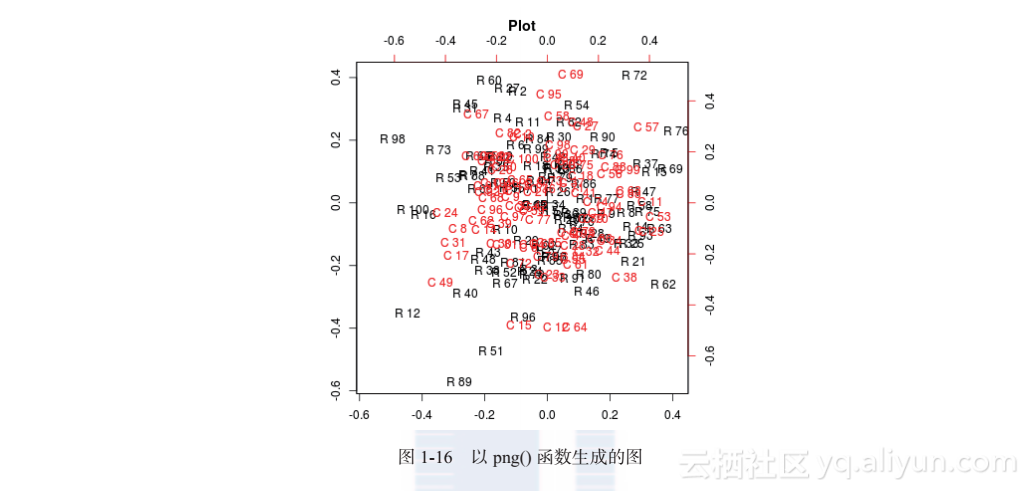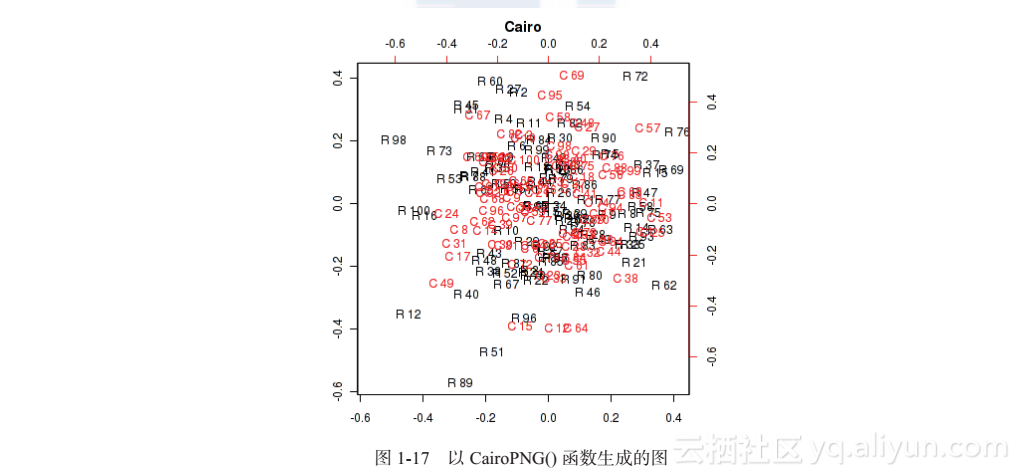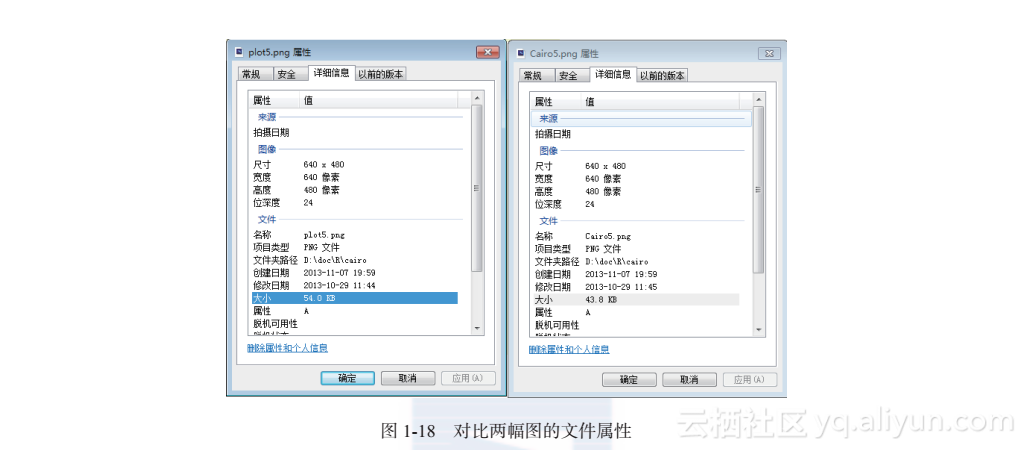SVG图形输出代码为：

> svg(file="plot-svg5.svg",width=6,height=6)
> biplot(corresp(m, nf=2), main="Plot-svg")
> dev.off()

> CairoSVG(file="Cairo-svg5.svg",width=6,height=6)
> biplot(corresp(m, nf=2), main="Cairo-svg")
> dev.off()【转载】如何用开源工具进行Multi-Cloud的自动化资源架构和变更？

2526 0【百度地图API】如何快速创建带有标注的地图？——快速创建地图工具+如何标注商家

1093 0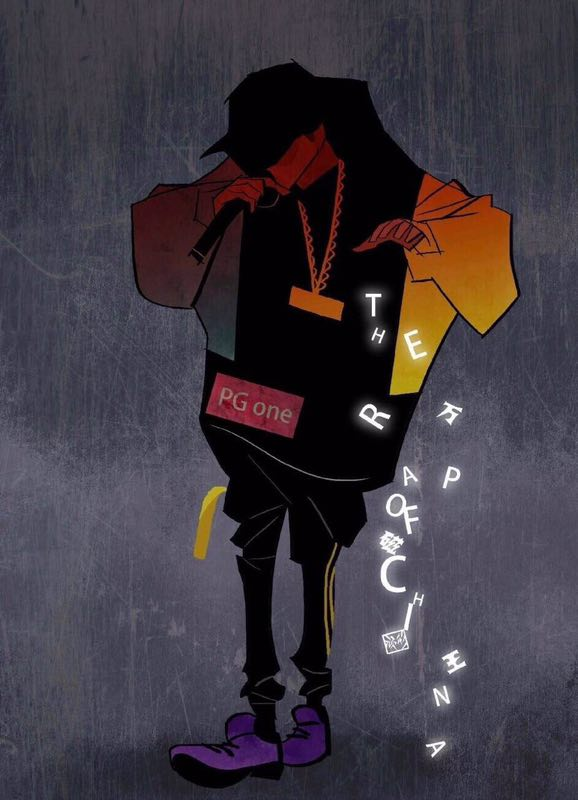1015 01434 0Java语言程序设计 上机实验6 掌握Java的图形用户界面编程，掌握布局管理器和事件的响应方法
Java语言程序设计 上机实验6 实验目的： 掌握Java的图形用户界面编程，掌握布局管理器和事件的响应方法。 实验内容：标(*)为选做内容 输入两个整数，分别进行加、减、乘、除等算术运算，界面如下图所示。
1082 0HanLP几乎实现了所有我们需要的繁简转换方式，并且已经封装到了HanLP中，使得我们可以轻松的使用，而分词器中已经默认支持多种繁简格式或者混合。这里我们不再做过多描述。
1397 0681 0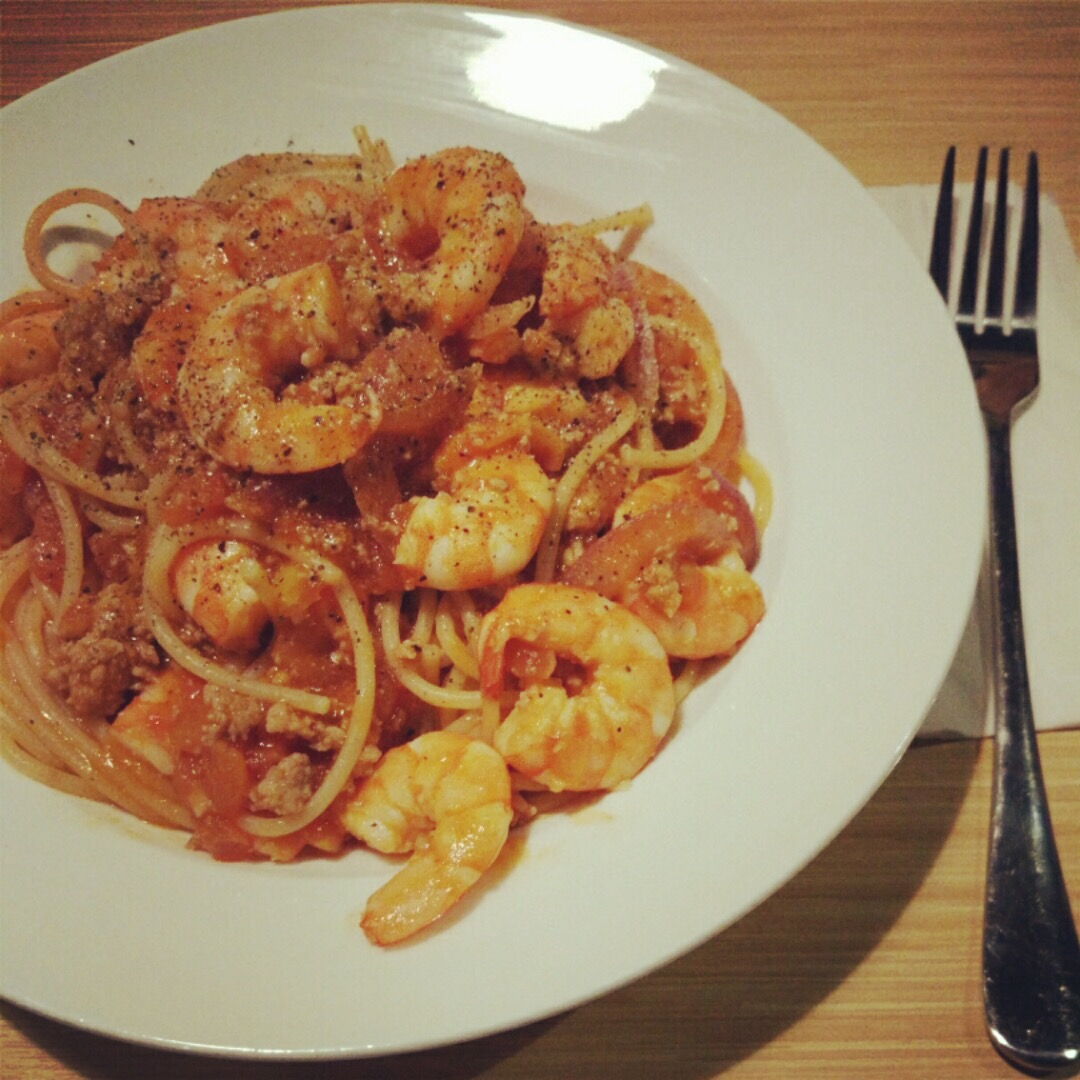2017年，大数据工程师应该如何充实自己的专业工具箱
2069 0C语言写一个GTK的二维码图形界面生成器
683 0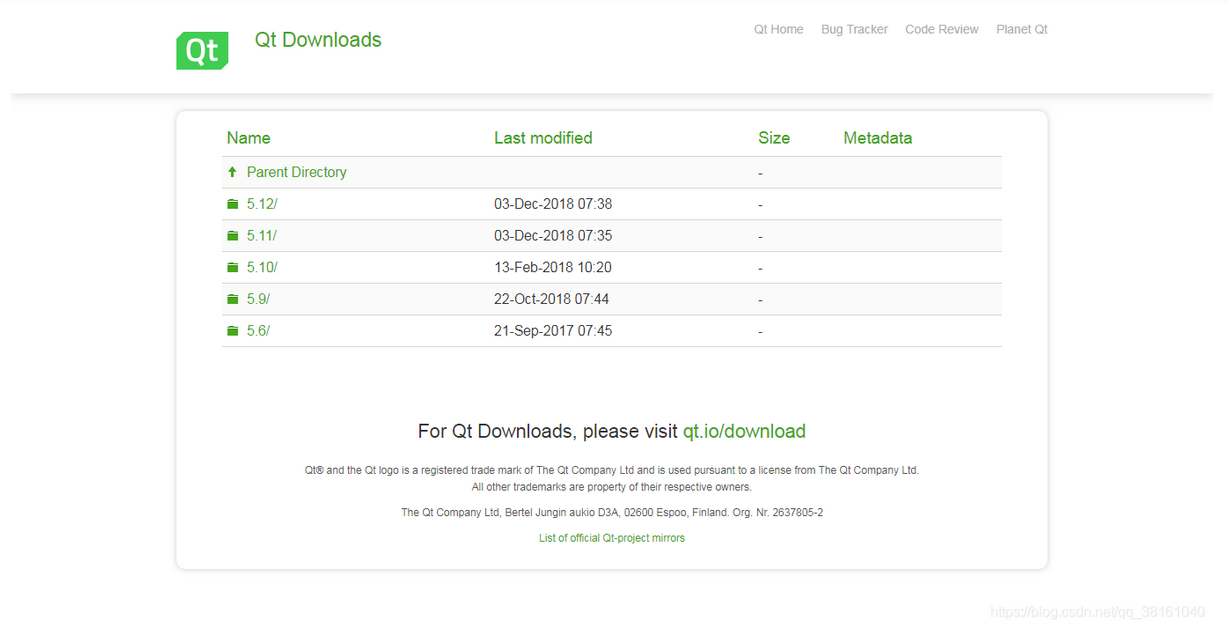PyQt5 图形界面-基础准备：安装Qt Creater、Qt Designer工具，使用Qt Designer设计界面，安装PyQt5工具库
PyQt5 图形界面-基础准备：安装Qt Creater、Qt Designer工具，使用Qt Designer设计界面，安装PyQt5工具库
38 0
10059

0

+ 订阅

JS零基础入门教程（上册）